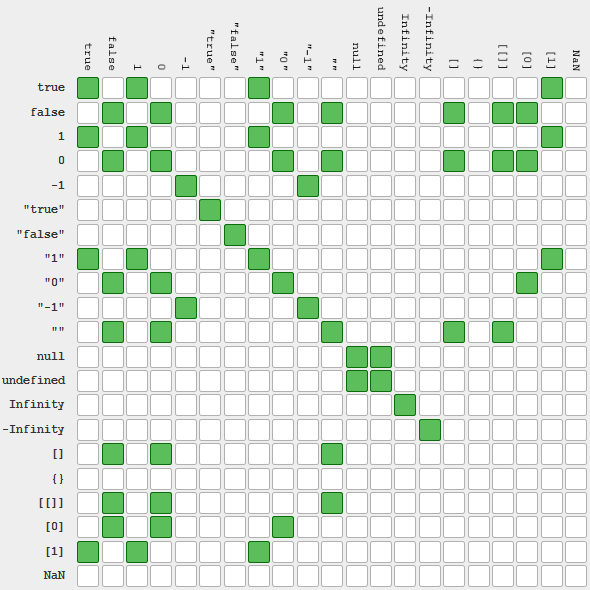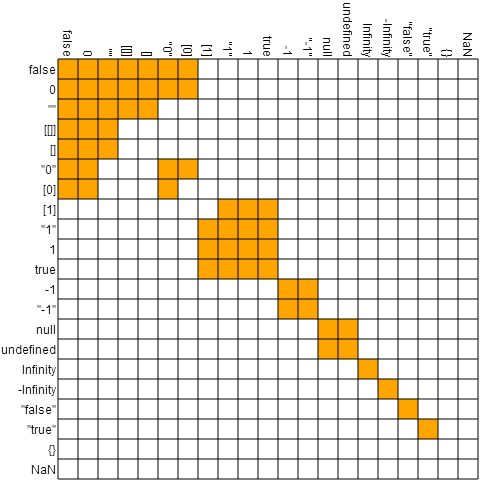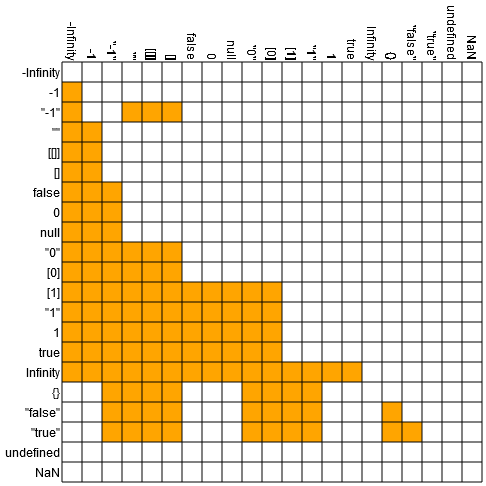# Don't Make Javascript Equality Look Worse Than It Is

27 Mar 2014

Every now and then someone posts a table showing what values are equivalent according to javascript's == operator, like this recent example. Then they point out how disorganized the table is.

These posts are fundamentally right about == being poorly designed... but they make things look worse by failing to organize the table. For example, here's the table from the linked post:What a mess! But most of the mess is because of the order of values in the table.

By grouping equal values together, you get something more sensible:That looks a lot better. Now you can see where things make sense, where reference equality is clashing with value equality, where there's a lot of reaching going on to consider different things equal, and where transitivity is violated.

Organizing the table reveals the actual flaws of ==, instead of obscuring them behind flaws in the diagram itself.

Code

Below is the code I used to draw the organized table. It's also available as a jsfiddle.

Html:

<canvas id="drawCanvas" width="500" height="500" />


Javascript:

var cmp = function(v1, v2) { return v1 == v2; };
var vals = [
["false", function() { return false; }],
["0", function() { return 0; }],
['""', function() { return ""; }],
["[[]]", function() { return [[]]; }],
["[]", function() { return []; }],
['"0"', function() { return "0"; }],
["", function() { return ; }],
["", function() { return ; }],
['"1"', function() { return "1"; }],
["1",function() { return  1; }],
["true", function() { return true; }],
["-1", function() { return -1; }],
['"-1"', function() { return "-1"; }],
["null", function() { return null; }],
["undefined", function() { return undefined; }],
["Infinity", function() { return Infinity; }],
["-Infinity", function() { return -Infinity; }],
['"false"', function() { return "false"; }],
['"true"', function() { return "true"; }],
["{}", function() { return {}; }],
["NaN", function() { return NaN; }]
];

var canvas = document.getElementById("drawCanvas");
var ctx = canvas.getContext("2d");
var n = vals.length;
var r = 20; // diameter of grid squares
var p = 60; // padding space for labels

// color grid cells
for (var i = 0; i < n; i++) {
var v1 = vals[i]();
for (var j = 0; j < n; j++) {
var v2 = vals[j]();
var eq = cmp(v1, v2);
ctx.fillStyle = eq ? "orange" : "white";
ctx.fillRect(p+i*r,p+j*r,r,r);
}
}

// draw labels
ctx.fillStyle = "black";
var f = 12;
ctx.font = f + "px Helvetica";
for (var i = 0; i < n; i++) {
var s = vals[i];
var w = ctx.measureText(s).width;
ctx.save();
ctx.translate(p+i*r+r/2-f*0.4,p-w-2);
ctx.rotate(3.14159/2);
ctx.fillText(s, 0, 0);
ctx.restore();
}
for (var i = 0; i < n; i++) {
var s = vals[i];
var w = ctx.measureText(s).width;
ctx.fillText(s, p-w-2, p+i*r+r/2+f*0.4);
}

// draw grid lines
ctx.beginPath();
ctx.strokeStyle = "black";
for (var i = 0; i <= n; i++) {
ctx.moveTo(p+r*i, p);
ctx.lineTo(p+r*i, p+r*n);
ctx.moveTo(p, p+r*i);
ctx.lineTo(p+r*n, p+r*i);
}
ctx.stroke();


Summary

JavaScript's == operator is intransitive and loose, flaws that absolutely justify using === instead, but it's not as bad as some tables make it look.

Update

It's a lot harder to make the < operator's truth table make sense (js fiddle here):A comparison operator's truth table should look like a triangle, or stair case, when put in the right order. JS's has several holes and flecks, because of how coercion varies based on the context.

(It's also notable that the best ordering for the comparison table is not a particularly good ordering for the equality table.)

Discussion on Reddit